# A small point mass carrying some positive charge on it,

Question:

A small point mass carrying some positive charge on it, is released from the edge of a table. There is a uniform electric field in this region in the horizontal direction. Which of the following options then correctly describe the trajectory of the mass? (Curves are drawn schematically and are not to scale).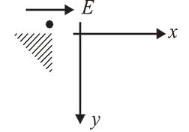1.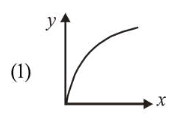2.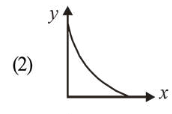3.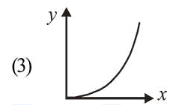4.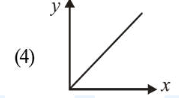Correct Option: , 4

Solution:

(4) Net force acting on the particle,

$\vec{F}=q E \hat{i}+m g \hat{j}$

Net acceleration of particle is constant, initial velocity is zero therefore path is straight line.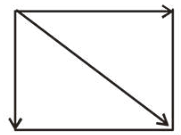$a_{x}=\frac{2 E}{m}$

$a y=g$

$a=\sqrt{\left(\frac{2 E}{m}\right)^{2}+g^{2}}$